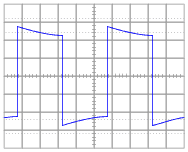# Oscilloscope probe principals

• Goodver
So at low frequencies the probe may have a longer time constant and at high frequencies the probe may have a shorter time constant. This allows us to have "infinite bandwidth" across the frequency range.f

#### GoodverAs far as I understand the problem is, because of inner capacitance of an oscilloscope, if we apply a rectangular signal on the screen we will see semi-sinusoidal one, and we want to handle this capacitance, that the scope shows correct signal. Right?

When we use a probe the circuit looks like a voltage devide:

http://www.elexp.com/tips/P_SCHPRB.GIF

By this voltage devider, as far as I can see, we reduce input voltage to oscilloscope by the factor of 10 (consider X10 probe).

My problems:

1. How this reduction of voltage removes the fluctuation on the 1 pic?
2. The devision of voltage by the factor of 10 will occur, as far as I understand, only at 0 frequency and infinite frequency, because in this case we can neglate resistors or capacitors, right? But if say frequency something like in the middle, then we have to consider all resistors and capacitors, which, I assume, will not give an exact devision by 10.

The answer to both questions will be clear when you see that Cp in the probe can be adjusted. Note that Rp and Cp constitute a zero, and the scope input a pole. The idea is the Cp in the probe is adjusted so its time constant (RpCp) equals the time constant of the scope input (RinCin). In this case, the pole and the zero can cancel and the probe gives you "infinite bandwidth". So the capacitors cancel out and the division is exactly 10 across the range.

This removes the fluctuation in the pic because the bandwidth is higher. If we just connect a resistive probe then the signal sees a low-pass filter which rounds the edges like in the picture.

"infinite bandwidth" is in quotes because of course parasitics will limit the performance of this system.

Good answer by analogdesign. You may also find the Textronix 'scope probe primer document useful:

http://www.tek.com/learning/probes-tutorialThank you! So the idea is to achieve equal time constant that the ratios between impedances of the scope and the probe stays the same, thus output voltage does not change